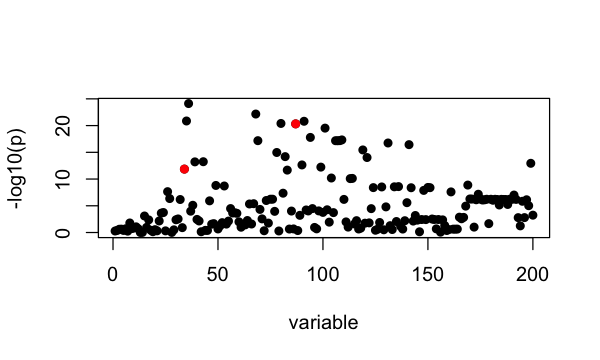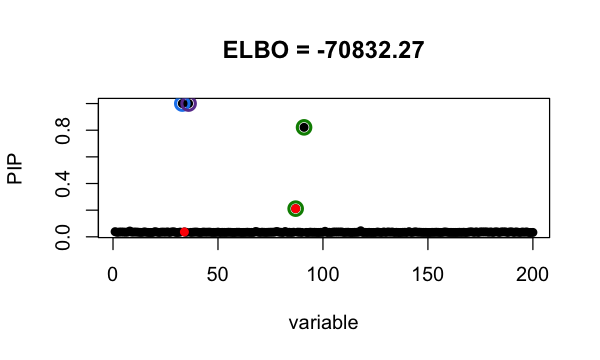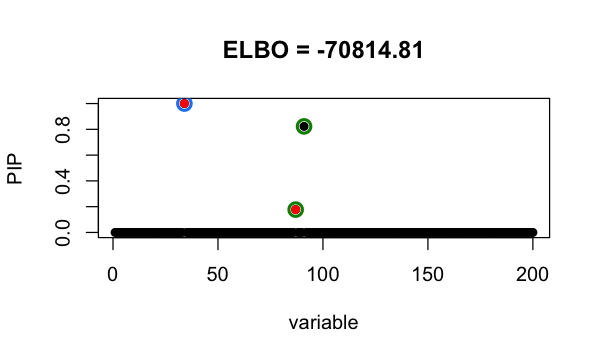# Refine SuSiE model

#### 2021-06-07

In this vignette, we demonstrate a procedure that helps SuSiE get out of local optimum.

We simulate phenotype using UK Biobank genotypes from 50,000 individuals. There are 200 SNPs. It is simulated to have exactly 2 non-zero effects at 34, 87.

library(susieR)
data('FinemappingConvergence')
b = FinemappingConvergence$true_coef susie_plot(FinemappingConvergence$z, y = "z", b=b)The strongest marginal association is a non-effect SNP.

Since the sample size is large, we use sufficient statistics ($$X^\intercal X, X^\intercal y, y^\intercal y$$ and sample size $$n$$) to fit susie model. It identifies 2 Credible Sets, one of them is false positive. This is because susieR get stuck around a local minimum.

fitted <- with(FinemappingConvergence,
susie_suff_stat(XtX = XtX, Xty = Xty, yty = yty, n = n))
susie_plot(fitted, y="PIP", b=b, main=paste0("ELBO = ", round(susie_get_objective(fitted),2)))Our refine procedure to get out of local optimum is

1. fit a susie model, $$s$$ (suppose it has $$K$$ CSs).

2. for CS in $$s$$, set SNPs in CS to have prior weight 0, fit susie model –> we have K susie models: $$t_1, \cdots, t_K$$.

3. for each $$k = 1, \cdots, K$$, fit susie with initialization at $$t_k$$ ($$\alpha, \mu, \mu^2$$) –> $$s_k$$

4. if $$\max_k \text{elbo}(s_k) > \text{elbo}(s)$$, set $$s = s_{kmax}$$ where $$kmax = \arg_k \max \text{elbo}(s_k)$$ and go to step 2; if no, break.

We fit susie model with above procedure by setting refine = TRUE.

fitted_refine <- with(FinemappingConvergence,
susie_suff_stat(XtX = XtX, Xty = Xty, yty = yty,
n = n, refine=TRUE))
susie_plot(fitted_refine, y="PIP", b=b, main=paste0("ELBO = ", round(susie_get_objective(fitted_refine),2)))With the refine procedure, it identifies 2 CSs with the true signals, and the achieved evidence lower bound (ELBO) is higher.

## Session information

Here are some details about the computing environment, including the versions of R, and the R packages, used to generate these results.

sessionInfo()
# R version 3.6.2 (2019-12-12)
# Platform: x86_64-apple-darwin15.6.0 (64-bit)
# Running under: macOS Catalina 10.15.7
#
# Matrix products: default
# BLAS:   /Library/Frameworks/R.framework/Versions/3.6/Resources/lib/libRblas.0.dylib
# LAPACK: /Library/Frameworks/R.framework/Versions/3.6/Resources/lib/libRlapack.dylib
#
# locale:
#  en_US.UTF-8/en_US.UTF-8/en_US.UTF-8/C/en_US.UTF-8/en_US.UTF-8
#
# attached base packages:
#  stats     graphics  grDevices utils     datasets  methods   base
#
# other attached packages:
#  ggplot2_3.3.0        microbenchmark_1.4-7 Matrix_1.2-18
#  L0Learn_1.2.0        susieR_0.11.42
#
# loaded via a namespace (and not attached):
#   Rcpp_1.0.5       pillar_1.4.3     compiler_3.6.2   plyr_1.8.5
#   highr_0.8        tools_3.6.2      digest_0.6.23    evaluate_0.14
#   lifecycle_0.1.0  tibble_2.1.3     gtable_0.3.0     lattice_0.20-38
#  pkgconfig_2.0.3  rlang_0.4.5      yaml_2.2.0       xfun_0.11
#  withr_2.1.2      stringr_1.4.0    dplyr_0.8.3      knitr_1.26
#  grid_3.6.2       tidyselect_0.2.5 reshape_0.8.8    glue_1.3.1
#  R6_2.4.1         rmarkdown_2.3    mixsqp_0.3-46    irlba_2.3.3
#  farver_2.0.1     reshape2_1.4.3   purrr_0.3.3      magrittr_1.5
#  scales_1.1.0     htmltools_0.4.0  assertthat_0.2.1 colorspace_1.4-1
#  stringi_1.4.3    munsell_0.5.0    crayon_1.3.4